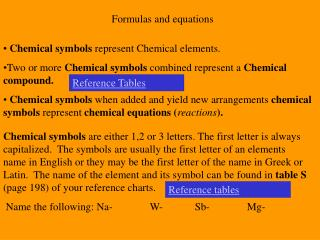DownloadDownload PresentationFormulas and equations

# Formulas and equations

Télécharger la présentation## Formulas and equations

- - - - - - - - - - - - - - - - - - - - - - - - - - - E N D - - - - - - - - - - - - - - - - - - - - - - - - - - -
##### Presentation Transcript

1. Formulas and equations • Chemical symbols represent Chemical elements. • Two or more Chemical symbols combined represent a Chemical compound. • Chemical symbols when added and yield new arrangements chemical symbols represent chemical equations (reactions). Reference Tables Chemical symbols are either 1,2 or 3 letters. The first letter is always capitalized. The symbols are usually the first letter of an elements name in English or they may be the first letter of the name in Greek or Latin. The name of the element and its symbol can be found in table S (page 198) of your reference charts.  Name the following: Na- W- Sb- Mg- Reference tables

2. Diatomic Molecules are molecules that do not exist in nature as one element. They need to be paired with other like elements. Nitrogen, Bromine, Fluorine, Iodine, Oxygen, Chlorine and Hydrogen. All these exist in nature combined as two. And have the subscript of 2. Example: H2 is the formula for hydrogen. Never Bring Fat Idiots On Climbing Hikes

3. Formulas tell the Qualitative and Quantitative information about a compound. Quantitative (represented by a subscript)is the numerical value assigned to each element. Qualitative is the type of element found in each compound (Metal or non-metal). Subscripts indicate the number of a particular atom in a compound. If the subscript is outside a set of parentheses all of the atoms and their subscripts within those parentheses are multiplied by the subscript. Counting atoms quiz

4. Empirical Formulas- is the smallest whole number ratio that a compound can have. Empirical formulas can be for Ionic compounds or Molecular compounds. Ionic compounds are formed from a metal and a non-metal. Electrons are transferred from the metal to the nonmetal In an Ioniccompound. Molecular compounds are formed between two non-metals. Electrons are shared by the atoms in a molecular compound. Ionic Formulas Molecular formulas Empirical Formulas for molecular compounds can be actual or they may be the lowest common denominator for the compound. Example : What is the empirical formula for C6H12O6 Empirical formulas

5. Atoms have an equal number of protons and electrons and are electrically neutral. Ions- Can either gain electrons and become negative in charge (Anions) or lose electrons and become positive in charge (Cations). Ions with a + or – 1 charge may be written with out the number. Anions are usually nonmetals and Cations are usually Metals.Positive ions are attracted toward Negative ions to form ionic compounds. Ions want to be neutral so positives want to be balanced by the same # of negatives. Chemical formulas Ionic compounds

6. Poly atomic ions can be found in reference table E. These are a group of molecules which are covalently bonded but behave as if they were a single anion. Ionic Quiz Reference tables What is an example of a positive poly atomic ion? NH4+

7. Coefficients indicate the total # of units and it applies to every element in the compound. They also represent the # of moles.

8. Forensic balancing Balanced equations

9. Synthesis Decomposition

10. Single replacement Double Replacement Sample Problems Answers